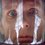# Playing with Integrals: Riemann sums

When we introduce definite integral in Mathematics we usually start with very formal definition -- the Riemann Sum. Many of us consider it quite dull and boring, because it doesn't directly help in the pen-and-paper calculation (but it's basis for computer calculation) of integrals. However, understanding this definition and taking advantage of it is extremelly useful when tackling an Olympiad problem.

The Riemann sum of a function $f:D\rightarrow\mathbb{R}$ over the closed interval $I=[a,b]\subset D$ with partition $P=\{[x_0,x_1),...,[x_{n-1},x_n]\}$is $S=\sum_{i=1}^n f(\xi_i)(x_i-x_{i-1}),\, \xi\in[x_{i-1},x_i].$ Where $a=x_0 and $\xi_i$ is a random number from the interval $[x_{i-1},x_i]$.

Some Riemann sums have special names, e.g. the left Riemann sum is a sum where $\xi_i=x_{i-1}$ and the right Riemann sum is a sum where $\xi_i=x_i$. The last two types of sums I want to discuss are upper and lower Riemann sums. To get them we subtitute $f(\xi_i)$ in our sum with $\sup/\inf \{f(x), x\in[x_{i-1},x_i]\}$. All this definitons help us finaly derive the notion of Riemann integral $\int^b_a f(x)\,dx=\lim_{n\to\infty}\left(\sum_{i=1}^n f(\xi_i)(x_i-x_{i-1})\right).$Note by Nicolae Sapoval
6 years, 10 months ago

This discussion board is a place to discuss our Daily Challenges and the math and science related to those challenges. Explanations are more than just a solution — they should explain the steps and thinking strategies that you used to obtain the solution. Comments should further the discussion of math and science.

When posting on Brilliant:

• Use the emojis to react to an explanation, whether you're congratulating a job well done , or just really confused .
• Ask specific questions about the challenge or the steps in somebody's explanation. Well-posed questions can add a lot to the discussion, but posting "I don't understand!" doesn't help anyone.
• Try to contribute something new to the discussion, whether it is an extension, generalization or other idea related to the challenge.

MarkdownAppears as
*italics* or _italics_ italics
**bold** or __bold__ bold
- bulleted- list
• bulleted
• list
1. numbered2. list
1. numbered
2. list
Note: you must add a full line of space before and after lists for them to show up correctly
paragraph 1paragraph 2

paragraph 1

paragraph 2

[example link](https://brilliant.org)example link
> This is a quote
This is a quote
    # I indented these lines
# 4 spaces, and now they show
# up as a code block.

print "hello world"
# I indented these lines
# 4 spaces, and now they show
# up as a code block.

print "hello world"
MathAppears as
Remember to wrap math in $$ ... $$ or $ ... $ to ensure proper formatting.
2 \times 3 $2 \times 3$
2^{34} $2^{34}$
a_{i-1} $a_{i-1}$
\frac{2}{3} $\frac{2}{3}$
\sqrt{2} $\sqrt{2}$
\sum_{i=1}^3 $\sum_{i=1}^3$
\sin \theta $\sin \theta$
\boxed{123} $\boxed{123}$